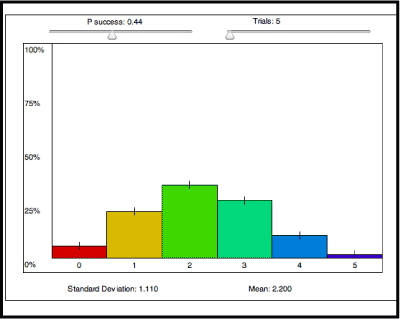# Functions, Statistics, and Trigonometry

Main goal: The goal of Functions, Statistics, and Trigonometry is to present topics from these three areas in a unified way to help students prepare for everyday life and future courses in mathematics. Spreadsheet, graphing and CAS technology are employed to enable students to explore and investigate, and to deal with complicated functions and data.

Main theme I: This text extends student knowledge of linear, quadratic, exponential, logarithm, polynomial and trigonometric equations and functions, with a new focus on statistical modeling with these functions.

Main theme II: Statistics are introduced in this text in the ways that people who work in a variety of different disciplines use them. Major topics include the selection of statistical displays, the differences between population and sample statistics, statistical distributions with emphasis on binomial and normal distributions, and statistical inference in addition to the statistical modeling mentioned above.

Main theme III: Trigonometry is explored. Trigonometric functions are used in their two main roles: as functions that enable lengths of segments and measures of angles of figures to be determined, and as functions that model periodic phenomena. The work with trigonometry includes strong connections with the geometry, matrices, and complex numbers that students encountered in previous courses.Binomial Probability Applet

Main theme IV: The themes of statistics and trigonometry are integrated. By viewing statistical distributions as functions, properties of one idea can be applied to the other. A typical example is the transformation of graphs and its conceptual relative, the standardization of data. Modeling data by functions enables an examination of the distinguishing characteristics of the various types of functions that make them important.

Comparison between this and earlier editions: The statistical work has been rewritten with more emphasis on decision-making. The work with functions and trigonometry remains about the same as in previous editions.

Some distinctive lessons: Using statistics to solve a mystery: The Federalist papers (1-8); From New York to New Delhi (5-10); Designing simulations (6-7); Polynomial models (7-2); How much does a loan cost? (8-6); Is that coin fair? (10-8); The geometry of complex numbers (13-5).

### Contact

UCSMP
1427 East 60th Street
Chicago, IL 60637
T: 773-702-1130
F: 773-834-4665
ucsmp@uchicago.edu